### ELECTRICAL CIRCUIT

Fun with science > Knowledge Hub > Science > Physics > ELECTRICAL CIRCUIT

# ELECTRICAL CIRCUITWhat is an electrical circuit?

A connection that provides a path for the electricity to flow from the positive terminal to the negative terminal of the cell, is called an electrical circuit. For example, a bulb glows only when the positive terminal and the negative terminal of an electric cell are
connected to the two terminals of the bulb.

For an electric current to happen, there must be a circuit.

If the wires from the bulb are connected to the same terminal (either both positive or both negative), then the bulb does not glow.

Sometimes, the bulb may not glow even though the connections are properly made. This may be because the flow of electricity is incomplete as the filament in the bulb is broken.

CLOSED CIRCUIT

A circuit is a closed path or loop around which an electric current flows. A circuit is usually made by linking electrical components together with pieces of wire cable.

Below is a basic set of symbols that you may find on circuit diagrams.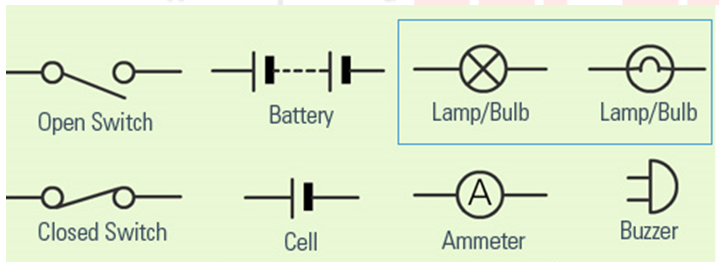SWITCH:
The switch is simply a small gap in the conductor where you can close or open the circuit. When the switch is closed, the circuit is closed and electricity flows.
When the switch is in the ‘OFF’ position, the circuit is incomplete. It is said to be open. No current flows through any part of the circuit. This is why a switch is sometimes called a circuit breaker.

You might have noticed that a glowing electric bulb become warm. Do you know why?

RESISTORS

Anything that uses some of the power from the cell is called a resistor.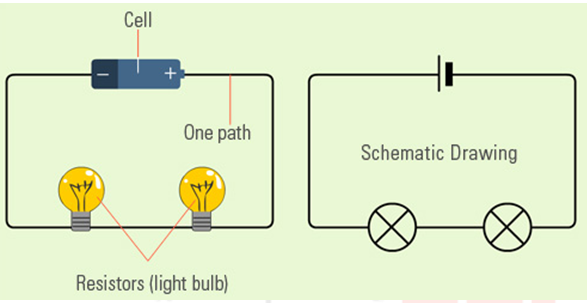Each time there is damage (break) in any one of the resistors the entire circuit will not function. For example, if one light bulb goes out, all the other lights will go off because the electricity path in the broken bulb is cut off.

CONDUCTORS:
These are usually copper wires. They make the path through which the electricity flows. One piece of the wire connects the current from the power source (cell) to the load. The other piece connects the load back to the power source.

The diagram below shows how a basic circuit looks like.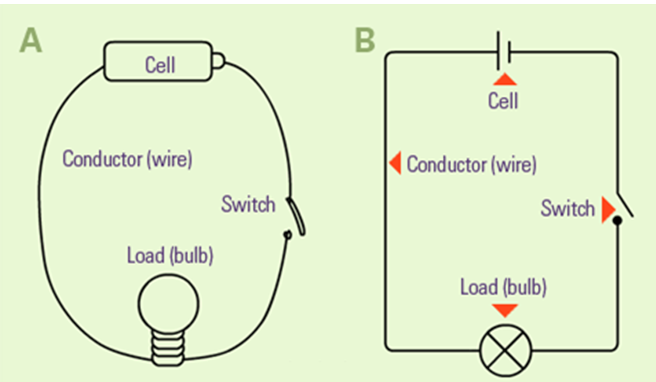There are two types of circuits namely Series Circuit and Parallel Circuit.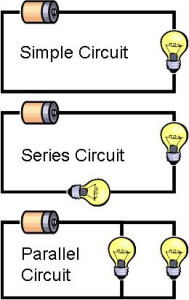SERIES/PARALLEL

SERIES CIRCUITS
A series circuit is one all of the current flows through each part of the circuit.

From one end of the cell (battery), the electrons move along one path with NO branches, through the resistors, to the other end of the cell. All the components in a series circuit are connected end-to-end. So, the current in the all components is same.

So, In a series circuit, every device must function for the circuit to be complete. One bulb burning out in a series circuit breaks the circuit.

Do you put Diwali lights on the trees at home during Diwali? If the lights are in a series circuit, one burned out bulb will keep all the lights off. That is one disadvantage of series circuits.

If there are many bulbs in a series circuit with a battery (cell), it is very likely that the light will be dimmer because many resistors are acting on the same voltage of power from the battery.

PARALLEL CIRCUIT

In a parallel circuit the current is divided into separate paths/branches. If the light bulbs are connected in parallel, the current flowing through the light bulbs combine to form the current flowing in the battery, In parallel circuits, each light has its own circuit, so all but one light could be burned out, and the last one will still function.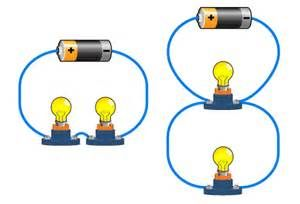NEVER PLAY WITH ELECTRICITY

BOOKS

We have our e-books published on Amazon for Grade 3 and Grade 4. The books serve as an important guide for Science Olympiads organized by SOF, Silverzone, Unified Council and others. Books are designed to help students understand key science concepts.

The key highlights of the book are:

·      Well explained topics

·      Use of diagrams and images for students to visualize

·      Test exercise after each chapter for self-assessment and evaluation

·      Interesting facts sections spread across the book